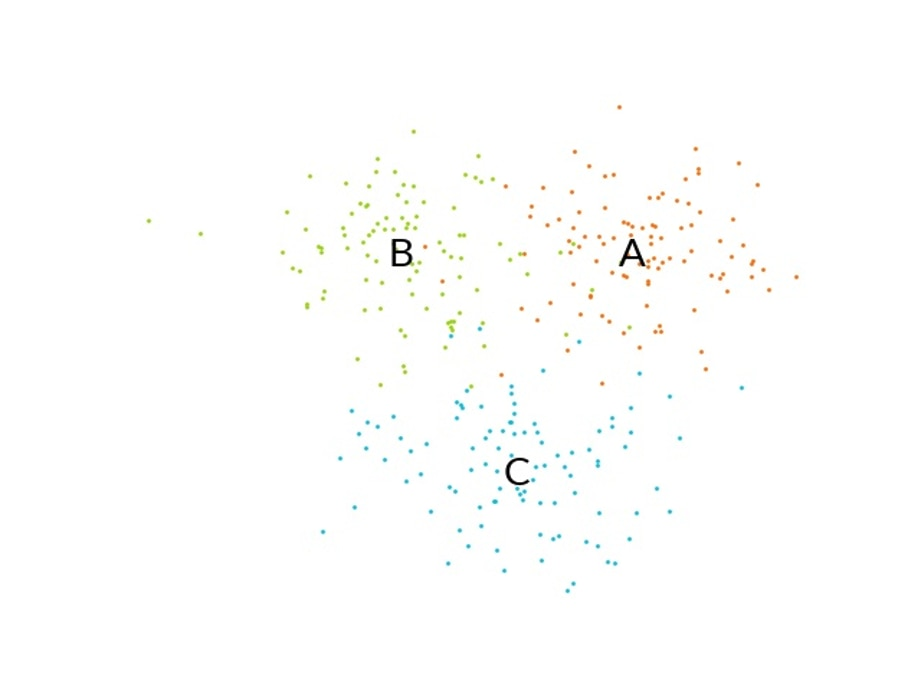## Train your Bayesian estimator with scikit-learn

In this section of the guide, we describe how to train the Bayesian classifier with scikit-learn and how to dump the parameters for use with CMSIS-DSP. The data generation and visualization parts of this activity are beyond the scope of this guide.

The file CMSIS/DSP/Examples/ARM/arm_bayes_example/train.py contains all the code for this example.

You can run this file to reproduce the results of this guide, and to generate the data and train the classifier.

In the example, there are three clusters: A, B, and C. The samples in each cluster are generated using a gaussian distribution.

The following image displays the three clusters of points:Figure 5: Three clusters of points: A, B, and C

The training of the Bayesian classifier is relying on the scikit-learn library. So, we must import GaussianNB from the sklearn.naive_bayes module.

Training requires some data. The random, numpy, and math Python modules are imported for the data generation part of this exercise.

The following Python code loads the required modules:

```from sklearn.naive_bayes import GaussianNB
import random
import numpy as np
import math```

The following code generates three clusters of points:

```# 3 cluster of points are generated
x1 = [1.5, 1] +  ballRadius * np.random.randn(NBVECS,VECDIM)
x2 = [-1.5, 1] + ballRadius * np.random.randn(NBVECS,VECDIM)
x3 = [0, -3] + ballRadius * np.random.randn(NBVECS,VECDIM)```

All the points and their classes are concatenated for the training.

Cluster A is class 0, cluster B is class 1, and cluster C is class 2.

The following code creates the array of inputs by concatenating the three clusters. This code also creates the array of outputs by concatenating the class numbers:

```# All points are concatenated
X_train=np.concatenate((x1,x2,x3))

# The classes are 0,1 and 2.
Y_train=np.concatenate((np.zeros(NBVECS),np.ones(NBVECS),2*np.ones(NBVECS)))```

The following code trains the Gaussian Naïve Bayes classifier on the input arrays that were just created:

```gnb = GaussianNB()
gnb.fit(X_train, Y_train)```

We can check the result by classifying a point in each cluster.

The following code checks that a point in cluster A is recognized as being in cluster A. The class number of cluster A is 0. This means that y_pred should be 0 when this code is executed:

```y_pred = gnb.predict([[1.5,1.0]])
print(y_pred)```

Now, we want to use this trained classifier with the CMSIS-DSP. For this, the parameters of the classifier must be dumped.

The CMSIS-DSP Bayesian classifier uses the instance structure that is shown in the following code. The parameters of this structure are needed by CMSIS-DSP and must be dumped from the Python script:

```typedef struct
{
uint32_t vectorDimension;  /**< Dimension of vector space */
uint32_t numberOfClasses;  /**< Number of different classes  */
const float32_t *theta;          /**< Mean values for the Gaussians */
const float32_t *sigma;          /**< Variances for the Gaussians */
const float32_t *classPriors;    /**< Class prior probabilities */
float32_t epsilon;         /**< Additive value to variances */
} arm_gaussian_naive_bayes_instance_f32;```

The parameters that are required can be dumped with following Python code:

```print("Parameters")
# Gaussian averages
print("Theta = ",list(np.reshape(gnb.theta_,np.size(gnb.theta_))))

# Gaussian variances
print("Sigma = ",list(np.reshape(gnb.sigma_,np.size(gnb.sigma_))))

# Class priors
print("Prior = ",list(np.reshape(gnb.class_prior_,np.size(gnb.class_prior_))))

print("Epsilon = ",gnb.epsilon_)```